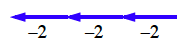### Home > CC2MN > Chapter 2 > Lesson 2.2.4 > Problem2-79

2-79.Find the value of each of the following expressions. Use a tile diagram or a number line to help you, if you need it.

1. $3(4)$

The parentheses used in this problem mean that you should multiply the numbers. If you are having trouble with multiplication, it may help to review problem 2-73.

1. $4+11+(-4)$

Remember that adding a negative number is just like taking steps backward, or subtracting.

1. $3.2(2)$

Think of the expression as asking how much $2$ groups of $3.2$ is.

1. $| ( - 2 ) + ( - 2 ) + ( - 2 ) |$

Again, adding negative numbers is like walking backwards. Does it help to think of the expression as a tightrope? The absolute value then removes the negative sign.The sum of the expression inside the absolute value symbols is $-6$, so the absolute value of the expression is $6$.

1. $| 2 ( - 7.5 ) |$

When you multiply a negative number and a positive number, the result is negative. See part (d) about the absolute value.

1. $(10\frac{1}{5})\left(-5\right)$

See the hint in part (e).

The product of this expression is $-51$.